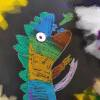{meta: 4/4} {meta: 速度: 71} {meta: 原调: 1=E} {meta: 选调: 1=C} ---------- [C] =节奏型= {start_of_tab} @ |-------x----------x--------| |-------x----------x--------| |----x----x-----x-----x-----| |---------------------------| |--x---------x--------------| |---------------------------| %----- ---- ----- ---- {end_of_tab} {rhythm: d (d (d u)) (d d)(d (d u))} {comment: Intro } [F]身 在 [G]他 乡 [Em]志 在 [Am]远 方 [Dm7]你的爱让[G]我 坚[C]强 [F]歌 声 [G]荡 漾 你[Em]为 我[Am]鼓 掌 [Dm7]沉浸在 爱[G]的 海[C]洋 {comment: Vrese 1 } 从[Dm7]不 敢想 到想 去做 到[G]做 到 我想的 事实[Dm7]证明我并不 像他 们想 象[G]的 那样 脆弱 我只是[F]需 要 一 盏 灯 一架[G]钢 琴一支 麦 克 [C]风 [C7] {comment: Chorus } [F]身 在 [G]他 乡 [Em]志 在 [Am]远 方 [Dm7]你的爱让[G]我 坚[C]强 [C7] [F]歌 声 [G]荡 漾 你[Em]为 我[Am]鼓 掌 [Dm7]沉浸在 爱[G]的 海[C]洋 {comment: Vrese 2} 曾经[Dm7]想象过 做一 名医 生[G]救死 扶 伤 也曾[Dm7]想象过做律师 做记者 做奥运冠军[G]但是都没有结果 因为我最 想[F]要的是一盏灯 一架[G]钢 琴一支 麦 克 [C]风 [C7] {comment: Chorus } [F]身 在 [G]他 乡 [Em]志 在 [Am]远 方 [Dm7]你的爱让[G]我 坚[C]强 [C7] [F]歌 声 [G]荡 漾 你[Em]为 我[Am]鼓 掌 [Dm7]沉浸在 爱[G]的 海[C]洋 {comment: M.solo} Ennn~~~~~~ [F] [G] [I] [C] [I] [F] [G] [I] [C] [I] [Bb] [I] [F] [C] [I] [Ab] [Bb] [I] [C] [I] {comment: Chorus } [F]身 在 [G]他 乡 [Em]志 在 [Am]远 方 [Dm7]你的爱让[G]我 坚[C]强 [C7] [F]歌 声 [G]荡 漾 你[Em]为 我[Am]鼓 掌 [Dm7]沉浸在 爱[G]的 海[C]洋 {comment: Chorus } [F]身 在 [G]他 乡 [Em]志 在 [Am]远 方 [Dm7]你的爱让[G]我 坚[C]强 [C7] [F]歌 声 [G]荡 漾 你[Em]为 我[Am]鼓 掌 [Dm7]沉浸在 爱[G]的 海[C]洋 {comment: Outro } [Dm7]沉浸在 爱[G]的 海 [C]洋 {title:未知名} {artist:未知名} {author:未知名}